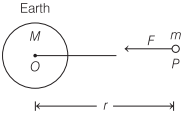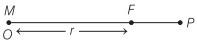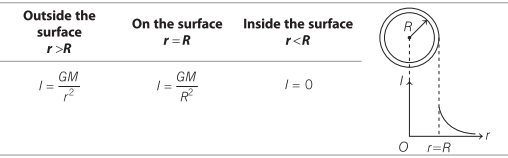# Gravitational Field in Physics | Definition, Formulas, Units – Gravitation

Gravitational Field Definition Physics:
The space in the surrounding of anybody in which its gravitational pull can be experienced by other bodies is called the gravitational field.

We are giving a detailed and clear sheet on all Physics Notes that are very useful to understand the Basic Physics Concepts.

## Gravitational Field in Physics | Definition, Formulas, Units – Gravitation

Gravitational Field Intensity:
The gravitational force acting per unit mass at any point in gravitational field is called intensity of gravitational field at that point. It is denoted by Eg or I.Eg or I = $$\frac{F}{m}$$

Intensity of gravitational field at a distance r from a body of mass M is given by

Eg or I = $$\frac{G M}{r^{2}}$$

It is a vector quantity and its direction is towards the centre of gravity of the body.

Gravitational Field Unit:
SI unit is N/m.

Gravitational Field Dimensional Formula:
Dimensional Formula is [LT-2].

### Gravitational Field Intensity for Different Bodies

1. Gravitational Field Intensity due to Point Mass:
Suppose a point mass M is placed at point O, then gravitational field intensity due to this point mass at point P is givenI = $$\frac{G M}{r^{2}}$$

2. Gravitational Field Intensity for Solid Sphere:3. Gravitational Field Intensity due to Spherical Shell:4. Gravitational Field Intensity due to Uniform Circular ring:Gravitation:
Have you ever thought, when we throw a ball above the ground level, why it returns back to the ground. It’s because of gravity. When a ball is thrown above the ground in the opposite direction, a gravitational force acts on it which pulls it downwards and makes it fall. This phenomena is called gravitation.

Learn relation between gravitational field and potential field, Kepler’s law of planetary, weightlessness of objects in absence of gravitation, etc.